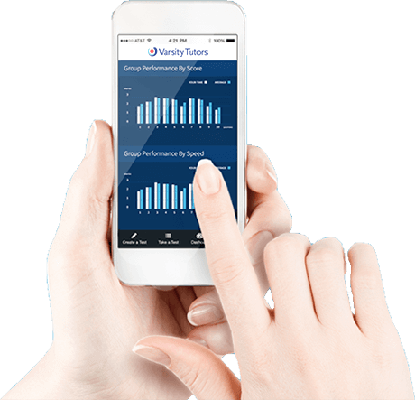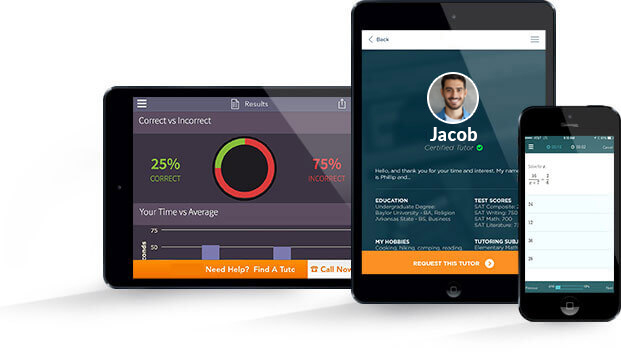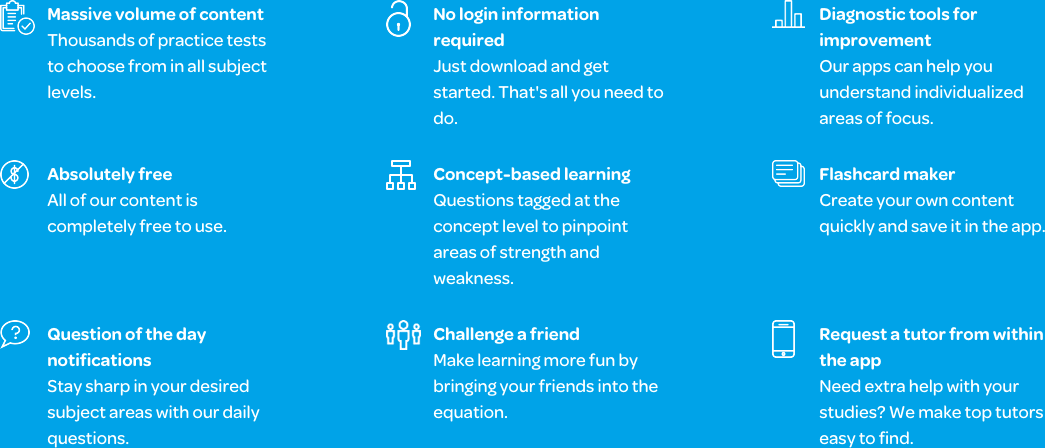# Varsity Tutors SAT II Math 2 mobile app### The Varsity Tutors SAT II Math 2 Mobile App

If you’re a university-bound student who wants to make a big impression, taking the SAT Subject Test for Math 2 (formerly known as the SAT II Math 2 Test) will showcase your proficiency and passion for higher-level math. You should consider taking the SAT Math 2 test if you have earned at least a B in classes through trigonometry and pre-calculus or calculus.

With Varsity Tutors’ free SAT Math 2 Subject Test app for iPhone, iPad, and Android devices, you can review the geometry, algebra, trigonometry, and calculus topics you’ll see on the test. The app covers data analysis and statistics, including quartiles and interquartile range, representing data, standard deviation, and mean, median, and mode. The app also includes material on functions and graphing linear, parametric, piecewise, recursive, quadratic, conic, and trigonometric functions. You will also find information on the properties of functions, such as maximum and minimum, slope, x- and y-intercept, and more.

Coordinate geometry consists of circles, ellipses, and hyperbolas, symmetry, transformations, and the distance and midpoint formulas -- all of which you must know. The 2-dimensional geometry section delves into analyzing figures, calculating area, diameter, radius, and circumference, as well as finding angles and perimeter. In 3-dimensional geometry, you will work with 3D axes and coordinates, faces, surface area, and volume.

Other topics covered on the SAT Math 2 Subject Test include mathematical relationships like absolute value, exponents and logarithms, matrices, and many others. Number theory will test your knowledge of irrational numbers, number sets, real and complex numbers, and many other types of numbers. Finally, you will find sections on single-variable algebra and trigonometry. Trigonometry deals with triangles and the laws of sines and cosines, as well as sine, cosine, tangent, and other topics specific to working with triangles.

The app provides numerous full-length, timed practice tests, which you can use to see where your strengths and weaknesses are. The questions are written to closely resemble the questions you will find on the actual test. Once you know where your weaknesses are, you can use the Learn by Concept section of the app to study a broad overview of a section. You can also drill down into specific subjects, like graphing piecewise and recursive functions, or recalling the law of sines.

The flashcards are arranged by concept, too, and present you with just one question at a time. You can use these to do a quick review if you only have a minute or two. You can even use the Flashcard Maker to create custom flashcards using your own images, audio, and text.

After you have studied your weak areas, take another practice test, and see how you have improved, and what areas still need a little work. You can keep track of the tests you have taken and track your progress with the app.

Download the free Varsity Tutors SAT Math 2 app from iTunes or the Google Play Store today to get started on mastering these advanced math concepts today!The SAT II Math II has been changed to the SAT Subject Test in Math Level II. The subject test is similar to the Math I exam, but is more complex, with trigonometry and precalculus material added. The test is designed to assess your abilities and interest in taking higher-level math courses, such as calculus, statistics, and others. If you are considering taking the Math II SAT Subject Test, you may be looking to fortify your college applications. To ensure that you have a higher chance of success, you should be skilled in the majority of advanced math concepts taught in high school. It is recommended that students who intend to take this test have two years of algebra, one year of geometry, and one year in precalculus and/or trigonometry.

The SAT Subject Test in Math II is a 60-minute, 50-question multiple-choice exam that scores between 200 and 800 points. To score highly, you must be able to analyze data to determine statistics and probabilities; perform geometric, algebraic, and trigonometric formulas; and use numbers and basic operations. You will be asked to prove yourself in terms of coordination, three-dimensional thinking, trigonometric skills, and more. It is important to work carefully through each problem to find the proper result, as the wrong answers tend to anticipate certain missed steps. As a result, time management is also an important aspect for a test taker to refine as part of their exam preparation.

The first section of the test covers numbers and operations, which accounts for 10 to 14 percent of the total score. You will be required to demonstrate your knowledge of operations that involve manipulating complex numbers, ratios, proportions, working with exponents, and others. In addition, you'll be tasked with proving your understanding of the order of numbers through sequencing, vectors, elementary number theory, and matrices. These are some of the more basic concepts you will be tested on during the first section of the SAT Math II Test.

The second section of SAT Math II is the algebra and functions portion of the test. You will need to be capable of providing a definition for the domain or range of a function, create your own graphs to reflect the function, perform basic algebraic equations to identify slopes, ranges, and more. You will have to prove you can work with various types of functions, as well as identify them based on their properties. You will need to be able to use periodic, inverse trigonometric, logarithmic, polynomial, linear, rational, piecewise, parametric, and recursive functions. This section of the test accounts for 48 to 52 percent of the overall exam score.

The third section of the exam focuses on your abilities in geometry and measurement. There are three larger concepts you will be tested in while working in this section of the Math II SAT Test: coordinate geometry, three-dimensional or “solid” geometry, and trigonometry.

The coordinate geometry questions will revolve around forming polar coordinates, symmetry, working with parabolas and lines, and evaluating ellipses and transformations. It is important that you understand how to solve problems that require you to analyze figures or shapes through complicated equations. In addition, you will need to have a deep understanding of the formation of the coordinate plane in order to complete the problems you will see on the test. The coordinate portion of the geometry and measurement section accounts for 10 to 14 percent of your total score.

For the three-dimensional questions, you'll be required to work with a variety of three-dimensional objects to find their surface area and volume. In addition, you'll need to recall the formulas for pyramids, prisms, cylinders, spheres, and cones, as well as to coordinate three-dimensional sets. You may be provided with an image to use, or you may need to visualize or draw your own. You will need to know the key formulas to identify the diagonal of a cube or rectangle, the surface area for a wide range of 3D shapes, and more. This portion makes up 4 to 6 percent of the exam total.

The trigonometry portion of this section counts for 12 to 16 percent of the exam. You will need to be able to use the laws of cosines, sines, and tangents to find characteristics of triangles. In addition, you'll be required to use formulas to measure radii and double angles. You may be tasked with solving complicated identities, equations, and measurements. It is important to know and understand the importance of sohcahtoa and the different identities, including the trigonometric, Pythagorean, double-angle, and half-angle identities.

The final section of the Math II SAT exam is the data analysis, probability, and statistics portion. It is worth 8 to 12 percent of the overall exam. You will be given a set of data, either in terms of quantities or measurements. From there, you will need to demonstrate your ability to derive the arithmetic mean, range, median, and mode. In addition, you need to have a solid understanding of probability. To answer probability questions, you will have to be able to understand the general formulas used to calculate these results, as well as how to identify the key information in a word problem. Furthermore, you may need to predict the probability of an event that won't occur. Associating the proper formula with the problem you are given is particularly important here. You will also be required to work with graphs, plots, and standard deviation to fully respond to the questions on the test. You may be tasked with problems that revolve around least squares regression, which require a knowledge of exponents, quadratics, and linear equations.

The SAT Subject Test in Math II should be taken as early as the end of your sophomore year if you have already taken precalculus or trigonometry by then. It is ideal to take the exam right after the your most recent trig or precalc course has completed so the material is fresh in your mind. Regardless, with the right combination of academic math experience and effort, you can prepare yourself for the SAT Subject Test in Mathematics II.